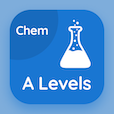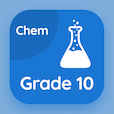A Level Courses

A Level Chemistry Practice Tests

A Level Chemistry Tests

The Book Chemical Formula and Equations Multiple Choice Questions (MCQ Quiz) PDF, Chemical Formula and Equations MCQs, download to study online a level chemistry courses. Practice Moles and Equations Multiple Choice Questions and Answers (MCQs), Chemical Formula and Equations quiz answers PDF for ACT prep classes. The eBook Chemical Formula and Equations MCQ App Download: atoms and molecules mass, solutions and concentrations, gas volumes, mole calculations test prep for accredited online colleges.

The MCQ: During a chemical reaction, atoms can not be PDF, "Chemical Formula and Equations MCQ" App Download (Free) with created only, destroyed only, converted, and created and destroyed choices for ACT prep classes. Study chemical formula and equations quiz questions, download Google eBook (Free Sample) for colleges that offer online courses.

## Chemistry: Chemical Formula and Equations MCQs

MCQ: During a chemical reaction, atoms can not be

A) created only
B) destroyed only
C) converted
D) created and destroyed

MCQ: The ions which are composed of more than one type of atom are called

A) ionic compounds
B) covalent compounds
C) compound ions
D) ionic ions

MCQ: The number of positive ions balances the number of negative charges so as to neutralize the compound and give the charge

A) one
B) unit
C) zero
D) negative

MCQ: The element which is never found completely pure in nature is

A) iron
B) copper
C) mercury
D) hydrogen

MCQ: The representation of a chemical reaction in shorthand way is via a

A) symbol equation
B) formula
C) covalent bonds
D) word equation

### Practice Tests: A Level Chemistry Exam Prep

Download A level Chemistry Quiz App, SAT Chemistry MCQ App, and 10th Grade Chemistry MCQs App to install for Android & iOS devices. These Apps include complete analytics of real time attempts with interactive assessments. Download Play Store & App Store Apps & Enjoy 100% functionality with subscriptions!